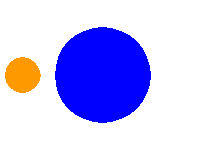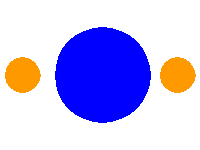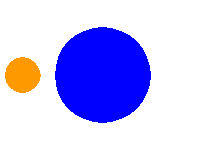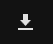# How to make SVG Loop Animation?

Loop Animation is useful to make a bird flying or a man walking etc.

In this article I will explane how to make SVG Loop Animation without using Java Script.

### SVG SMIL Animation

SVG stands for Scalable Vector Graphics. Many people think SVG is a vector-based static graphics format. SVG supports the animation which is called SMIL, Synchronized Multimedia Integration Language. SMILE animation works in all browsers except in Internet Explorer and Opera Mini. You can see the compatibility table on Can I Use.

### Simple animation example

The following is an example of an orange circle that moves from left to right in front of the blue circle.```  ```<svg>
<circle fill="blue" cx="150" cy="100" r="50"  />
<circle fill="orange" cy="100" r="20" >
<animate attributeName="cx" from="50" to="250"
dur="5s" repeatCount="indefinite" />
</circle>
</svg>
```
```
• `<animate>` element is used to animate `cx`. `cx` is the center position x of an orange circle.
• The `cx` is moving `from="50" to="250"` during five seconds(`dur="5s"`).
• `repeatCount="indefinite"` means the animation will be repeated indefinitely.

### Two circles moving around blue circle animation

In the next example, a new circle moving from right to left is added behind the blue circle. You will see two circles are moving around the blue circle.```  ```<svg>
<circle fill="orange" cy="100" r="20">
<animate attributeName="cx" from="250" to="50"
dur="5s"　repeatCount="indefinite" />
</circle>
<circle fill="blue" cx="150" cy="100" r="50" />
<circle fill="orange" cy="100" r="20">
<animate attributeName="cx" from="50" to="250"
dur="5s" repeatCount="indefinite" />
</circle>
</svg>
```
```

### How to repeat one circle moving around blue circle?

It was easy to make the animation that two circles are moving around the blue circle. But if you want to make the animation that one circle is moving around, you have to switch hide or display the circle alternately. You can't use `repeatCount="indefinite"`.

You can switch hide or display circle by using ID as follows.

1. Add ID to the both circles such as `id="o1", id="o2"`.
2. Add `begin` to each circle and define the start time of the animation.
3. Set the start time to the end time of the other circle animation(`o1.end、o2.end`).
```  ```<svg>
<circle fill="orange" cx="-50" cy="100" r="20">
<animate id="o1" begin="0;o2.end"
attributeName="cx" from="250" to="50" dur="5s" />
</circle>
<circle fill="blue" cx="150" cy="100" r="50" />
<circle fill="orange" cx="-50" cy="100" r="20">
<animate id="o2" begin="o1.end"
attributeName="cx" from="50" to="250" dur="5s" />
</circle>
</svg>
```
```The important point you have to think about is Stacking order.

• If the stacking order of the shapes doesn't change during the animation, you can use `repeatCount="indefinite"` to make loop animation.
• You can't use `repeatCount="indefinite"` if the stacking order will change during the animation. You have to use ID. But it will be complicated when the number of shapes becomes larger. You have to define many IDs when you change the stacking order of the shapes.

### Loop animation using dummy loop and relative time

I recommend you to define dummy loop at first, and set each start time by using relative time from the dummy loop as follows.

```  ```<svg>
<rect>
<animate id="o1" begin="0;o1.end" dur="10s"
attributeName="visibility" from="hide" to="hide"/>
</rect>
<circle fill="orange" cx="-50" cy="100" r="20">
<animate begin="o1.begin"
attributeName="cx" from="250" to="50" dur="5.05s"/>
</circle>
<circle fill="blue" cx="150" cy="100" r="50" />
<circle fill="orange" cx="-50" cy="100" r="20">
<animate begin="o1.begin+5s"
attributeName="cx" from="50" to="250" dur="5.05s"/>
</circle>
</svg>
```
```
• `<rect>` is a dummy object to define the loop time 10 seconds (`dur="10s"`).
• `id="o1" begin="0;o1.end"` means the rect 'o1' will start at 0 second and restart again at the end of animation.
• `attributeName="visibility" from="hide" to="hide"` means the rect will be hidden everytime.
• The start time of the orange circle is set relatively to `o1.begin` (as `begin="o1.begin"` and `begin="o1.begin+5s"`).
• In order to avoid blink in browser, the animation time is set to 5.05 seconds(`dur="5.05s"`).

## Example of SVG Loop Animation

Komori's SVG Animation is an example of the Loop Animation by using dummy loop and relative time. Almost browsers except IE can display this SMIL Animation.

### 2D Keyframe animation editor 9VAe

You can make SVG Loop Animation easily by using Openclipart and free software 9VAe.

Download (Clickin the following links) Download from Other How to Install
9va-win (Windows) 9va-win (Softnic) windows
9va-mac64 (macOS 10.10-)
9va-mac (Old Ver.10.4-10.9 is here)
9va-mac (Softnic) mac
9va-pi (Raspberry Pi) 9va-pi (Vector) pi
9va-pi (Ubuntu/Linux Mint/Xtra-PC (LinuxX86-32bit)) linux
PEAS motch! (Windows / Macintosh) Kids version)
Smartphone
Android 9VAe - Google Play How to make